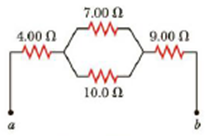Chapter 18, Problem 7P

Chapter
Section
Textbook Problem

(a) Find the equivalent resistance between points a and b in Figure P18.7. (b) Calculate the current in each resistor if a potential difference of 34.0 V is applied between points a and b.Figure P18.7

(a)

To determine
The equivalent resistance between the point a and b in the given figure P18.7.

Explanation

Given Info: The two parallel resistors is  R1P=7.00Ω and R2P=10.0Ω .

Explanation:

The two parallel resistors is R1P=7.00Ω and R2P=10.0Ω .

Formula to calculate the equivalent resistance of the two parallel resistors is,

1RP=1R1P+1R2P

• RP is the equivalent resistance ,
• R1P and R2P is the two parallel resistors,

Rewrite the above relation as RP .

RP=R1PR2PR1P+R2P

Substitute 7.00Ω for R1P and 10.0Ω for R2P .

RP=(7

(b)

To determine
The current through each resistor from the given figure P18.7.

Still sussing out bartleby?

Check out a sample textbook solution.

See a sample solution

The Solution to Your Study Problems

Bartleby provides explanations to thousands of textbook problems written by our experts, many with advanced degrees!

Get Started

Find more solutions based on key concepts